## How to model diffractive optics using the Binary 2 surface

Category: Product News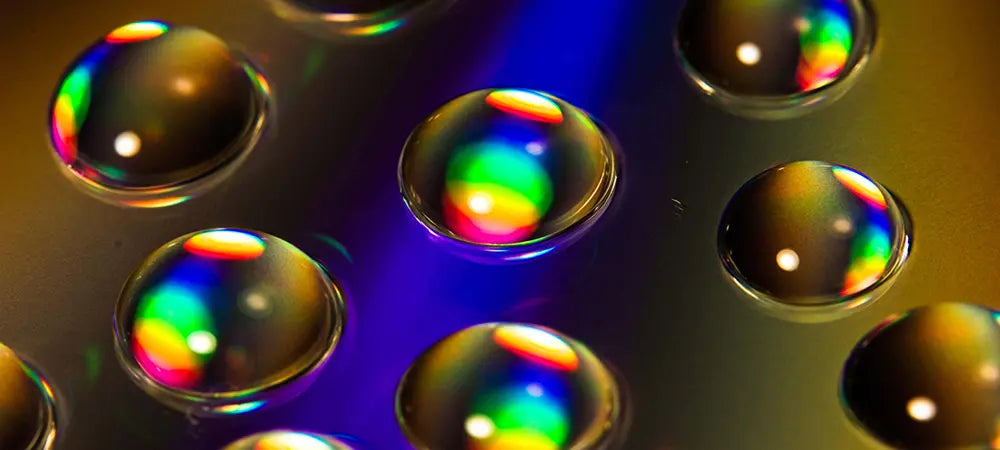Many surfaces in OpticStudio can have diffractive power in addition to refractive power. Diffractive power is independent of the index of refraction and the surface sag and changes the phase of the incoming rays.

Diffractive elements are commonly used in optical systems, and their implementation in OpticStudio Sequential Mode is important to understand for many optical designers. This blog post explains how diffractive surfaces are modelled in OpticStudio and includes a demonstration of using the Binary 2 surface type to model a diffractive optical element.

The Binary 2 surface

The diffractive power in a Binary 2 surface introduces continuous phase change across the surface according to the following formula:where the coefficients Ai are in units of radians.

Since the phase change is continuous across the surface, a Binary 2 surface represents an ideal binary diffractive optical element, in which the size of the binary (discrete) steps are infinitesimally small or at least very small compared to the wavelength.

Generally, the physical DOE modeled by the Binary 2 surface will have diffractive zones with varying period as a function of radial distance from the vertex, as shown in the following diagram.1 OpticStudio can calculate the radial coordinates of each diffraction zone, where the phase differs exactly by 2p from adjacent zones.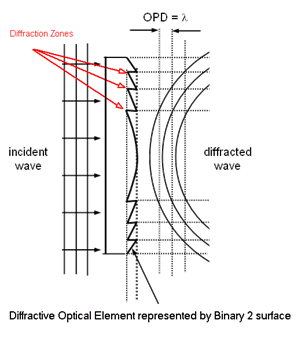The amount of phase in waves added by a Binary 2 surface at a particular radial coordinate is independent of wavelength. The wavelength-dependent optical path length (OPL) is given by

OPL = (Phase*wavelength) / (2p)

The following layout shows the chromatic effect due to a Binary 2 surface.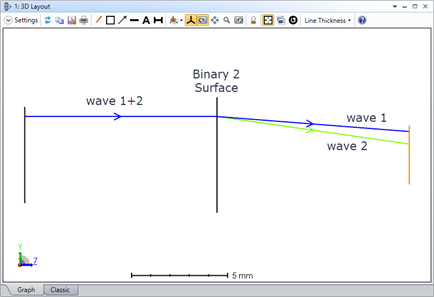Achromatization using Binary 2: Initial system setup

The Binary 2 surface is often used for achromatization. Consider a simple singlet lens in which the longer wavelength focuses at a larger Z distance from the lens than the short wavelength. A rotationally symmetric DOE such as Binary 2 surface can be used to minimize the longitudinal color.1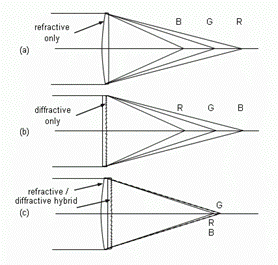Let's go through the process of designing the Binary 2 optics in the above figure. If you are not familiar with any of the steps in the exercise, please refer to the article “How to design a singlet lens."

We will design a DOE using the Binary 2 surface with diffraction order m=1 to correct longitudinal color. The completed design can be downloaded from the article attachments. First, set up the system properties and the singlet surfaces:

• Set the system length units to mm (System Explorer...Units)
• Set the system aperture as Entrance Pupil Diameter of 30 mm (System Explorer...Aperture)
• Set the wavelength to F, d and C. (System Explorer...Wavelengths); select F,d,C from the dropdown menu in the Wavelength Data window and hit Select Preset.
• Set surfaces in the Lens Data Editor with following parameters: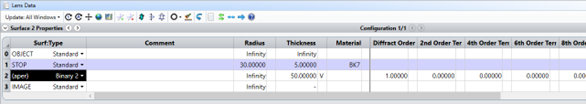The 3D layout now shows the singlet.Achromatization using Binary 2: Optimization of diffraction terms

Optimize the system for best focus using the RMS spot radius default Merit Function.After optimization, the variable in the editor, the thickness of surface #2, will be close to 51.608 mm.

The longitudinal aberration plot under Analysis...Miscellaneous...Longitudinal Color shows significant amount of longitudinal color aberration.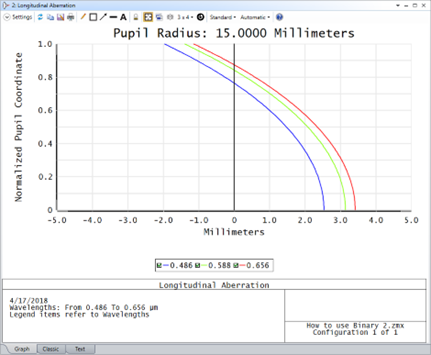We will now add some diffractive power to the Binary 2 surface.

In the Lens Data Editor, set the following parameter values and set the coefficients of p^2 and p^4 as variables:Re-optimize the system. Notice that the longitudinal color is now much smaller than before.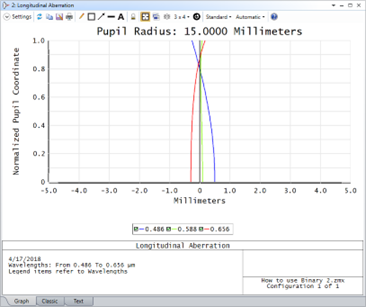Achromatization using Binary 2: Analyzing the surface phase profile

Now that we have the coefficients for the Binary 2 surface and know the phase profile, we need to calculate the radial coordinates of each 2*m*p diffraction zones to be used for the fabrication. The phase at each diffraction zones will differ exactly by +2*p or -2*p radian from the adjacent zones as shown in figure (a) of the image below.1

Run the macro “Phases.zpl” (found under Programming…ZPL Macros…Macro List) and specify surface 2 when prompted.The result shows that there are 246 zones with the last zone being at 14.94 mm from the vertex of the surface.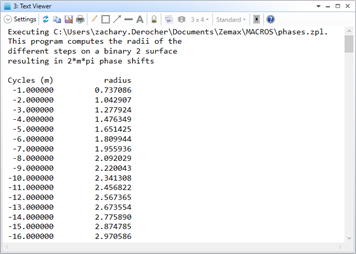The diagram below illustrates several different possibilities for the DOE surface profile.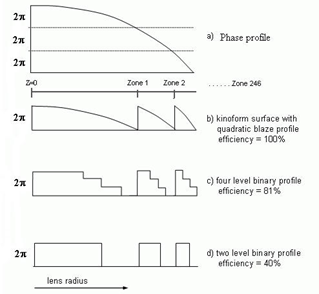Interested in learning more about OpticStudio optical design software capabilities?  Request a free trial today!

Author:
Nam-Hyong Kim

References

1. Fisher, Robert Edward., Biljana Tadic-Galeb, and Paul R. Yoder. Optical System Design. New York: McGraw-Hill Professional, 2008.
2. Battersby, Colin. "Zemax OpticStudio Tools for Diffractive Optics." Lecture.
3. Webinar: Tools for diffractive optics